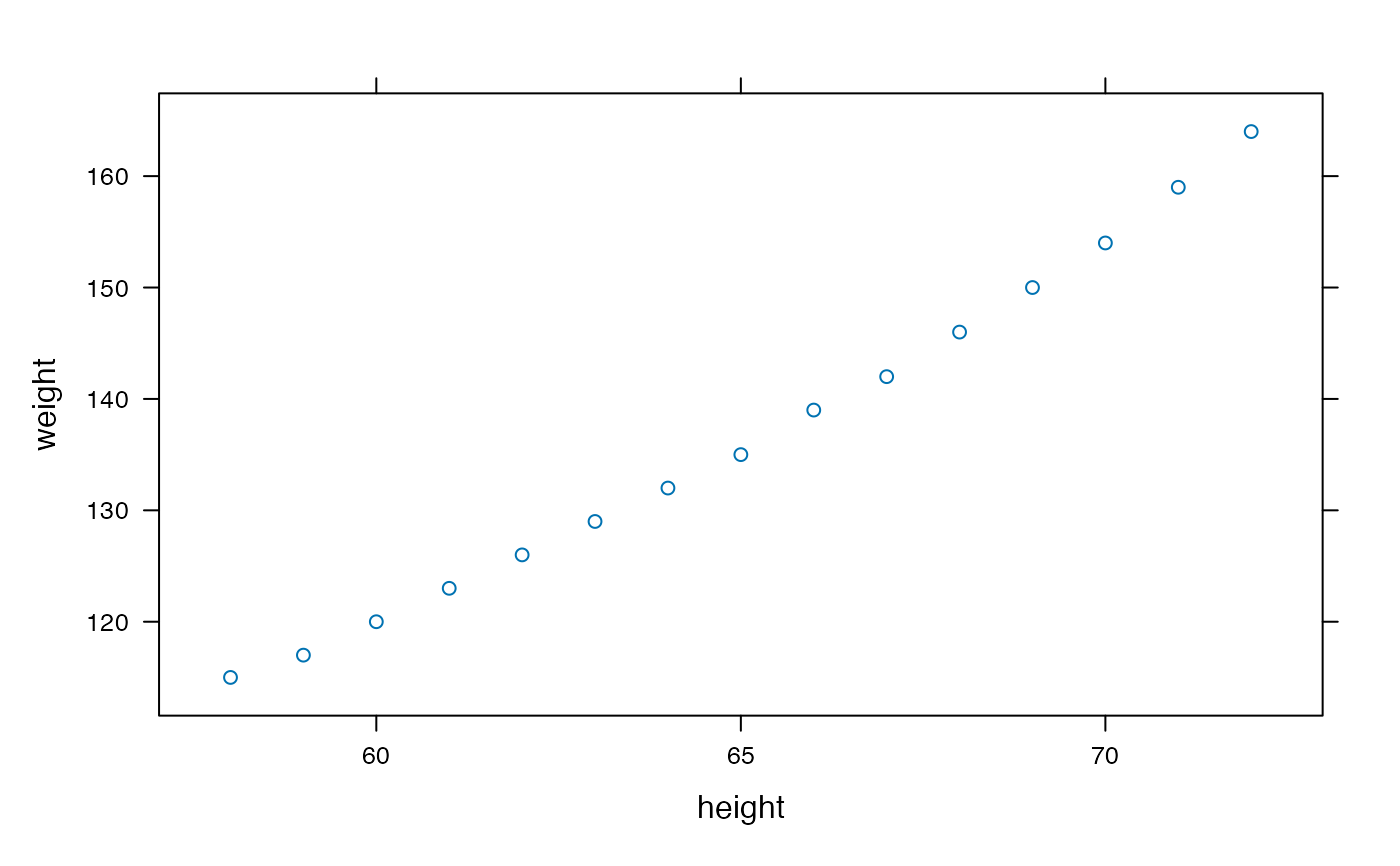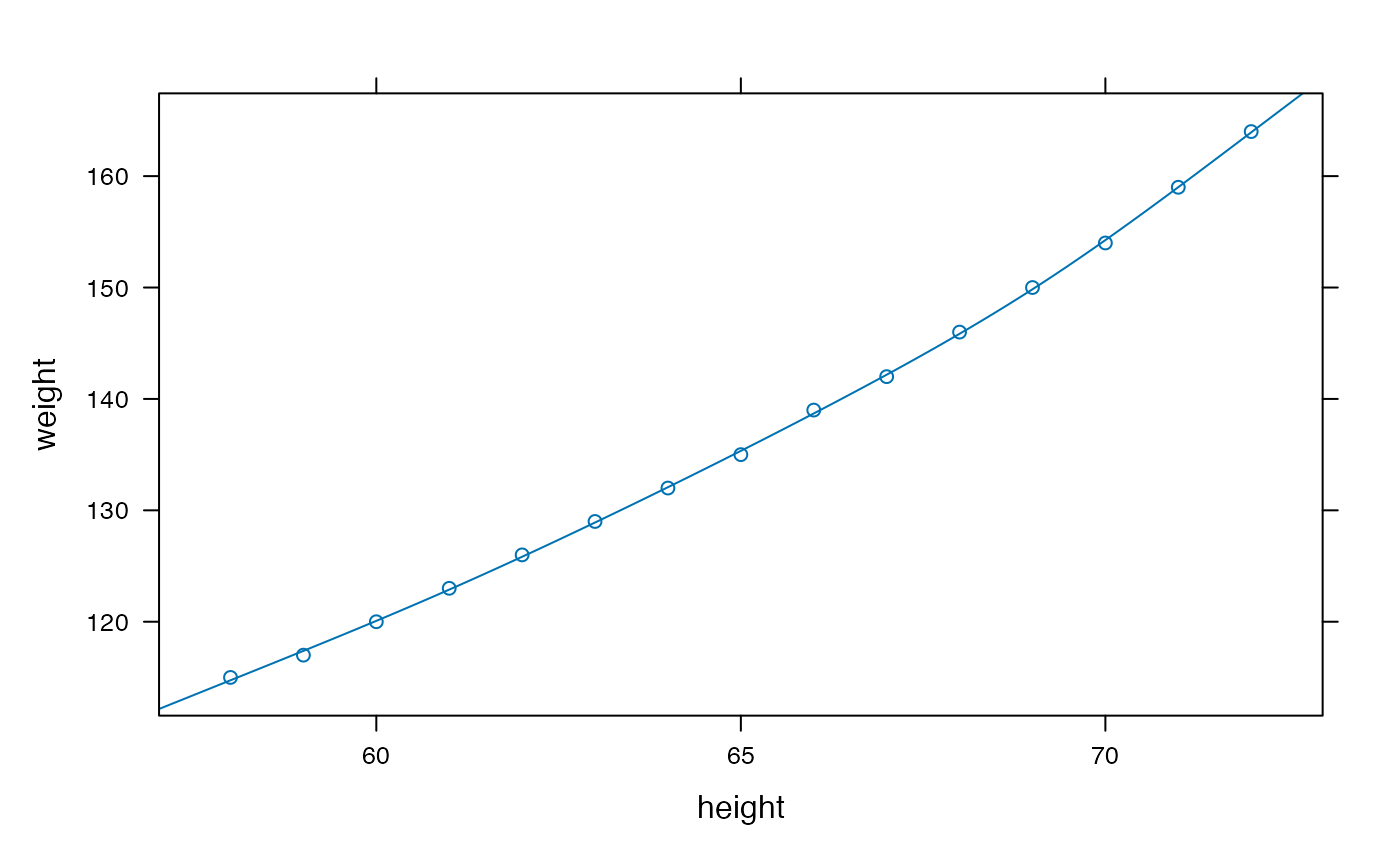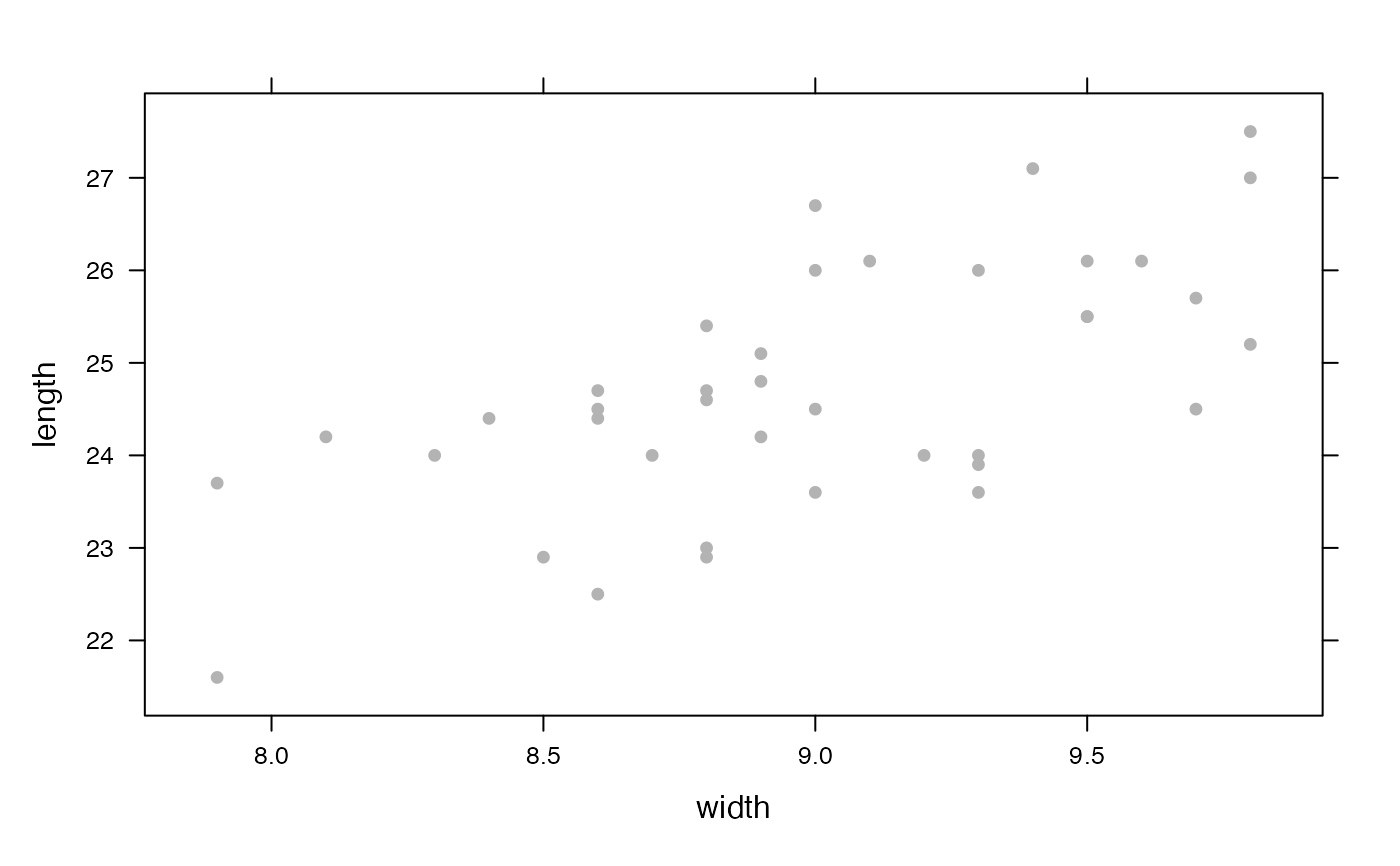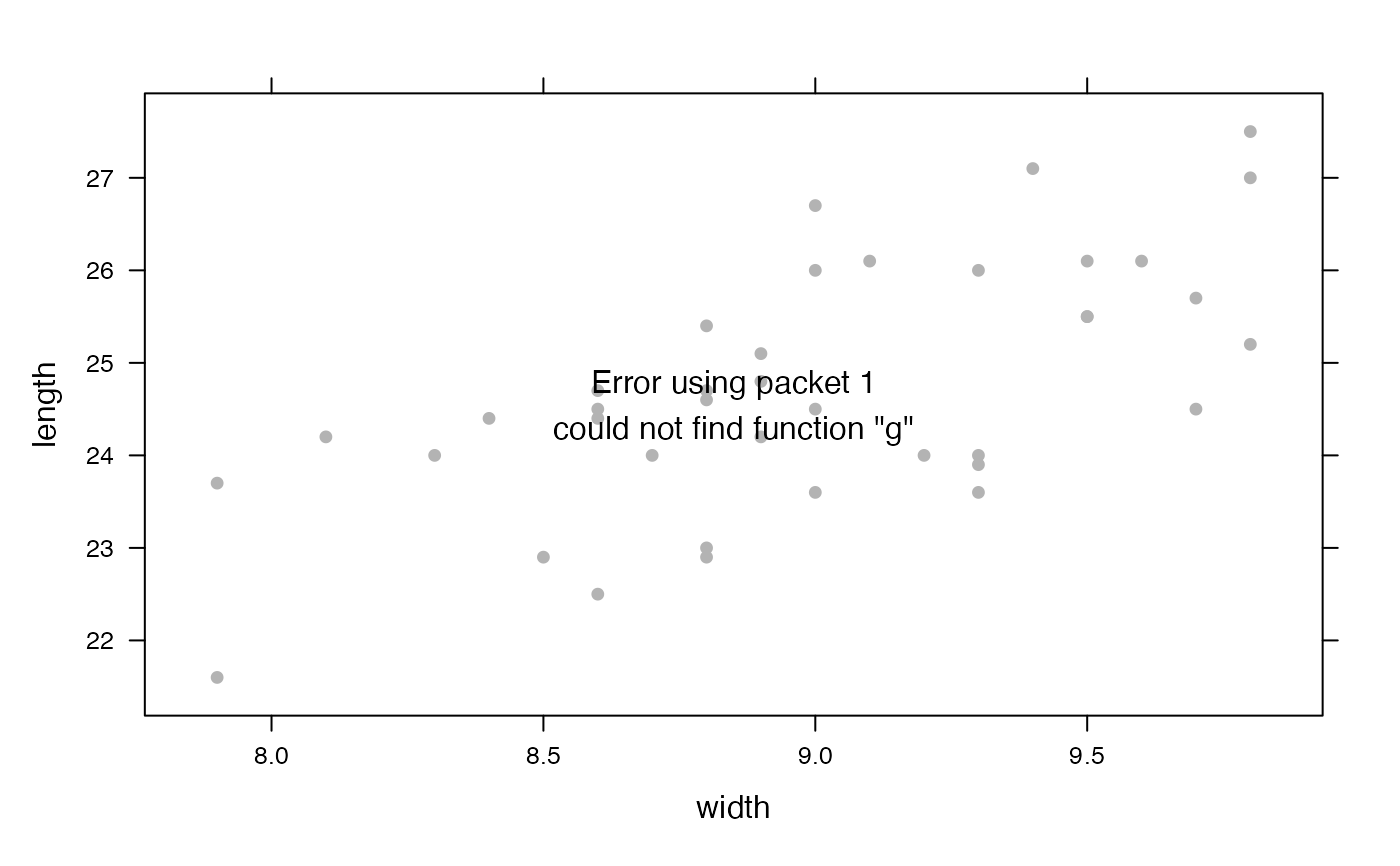These functions create mathematical functions from data, using splines.

fitSpline(
formula,
data = parent.frame(),
df = NULL,
knots = NULL,
degree = 3,
type = c("natural", "linear", "cubic", "polynomial"),
...
)

## Arguments

formula

a formula. Only one quantity is allowed on the left-hand side, the output quantity

data

a data frame in which formula is evaluated.

df

degrees of freedom (used to determine how many knots should be used)

knots

a vector of knots

degree

parameter for splines when type is "polynomial". 1 is locally linear, 2 is locally quadratic, etc.

type

type of splines to use; one of "linear", "cubic", "natural" (cubic with linear tails, the default), or "polynomial".

...

additional arguments passed to spline basis functions (ns() and bs()).

## Value

a function of the explanatory variable

bs() and ns() for the bases used to generate the splines.

## Examples

f <- fitSpline( weight ~ height, data=women, df=5 )
xyplot( weight ~ height, data=women )